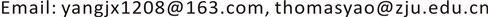1. 引言

2. 相关研究

3. 算法概述

1) 把多目标跟踪问题看作一个连续-离散的联合求解问题，实现数据关联和轨迹预测两大子问题的同步优化。将数据关联和轨迹预测这两个不同但紧密耦合的问题融合到统一的框架中实现递归式联合求解，并在结合微观社会行为分析的基础上利用标签代价函数对不合理的目标轨迹进行惩罚。

2) 在连续离散联合求解的框架基础上，增加目标组的行为建模，多个近邻的目标之间通常具有相似的位置，走向和速度等动力学特征，从而在一定的时域和空域中构成相对稳定的组。根据一种自底向上的分级聚类策略来测度场景中稳定存在的组，通过对组的外观和运动进行分析将有助于改善目标的数据关联和轨迹估计(图1)。

4. 连续离散联合求解算法4.1. 背景建模与运动分析目标检测

4.2. 连续离散问题联合求解

4.3. 连续轨迹模型

E f t e ( T ) = ∑ i = 1 N ( E f t e ( T i ) + E v t e ^ ( T i ) ) (1)

E f t e ( T i ) = ∑ t = s i e i ∑ j = 1 | D t | δ [ i − f d j t ] ⋅ c j t ⋅ ‖ p j t − T i ( t ) ‖ 2 (2)

E v t e ^ ( T i ) = ∑ s i − △ ≤ t < s i e i < t ≤ e i + △ ‖ v i t − T i ( t ) ‖ 2 (3)

‖ v i t − T i ( t ) ‖ 2 表示通过线性外推得到的虚拟位置V与轨迹上的对应点的欧式距离。Δ表示安全边界，一般取2。三次样条的目的是方便最大限度的能量最小化，在全局封闭的形式下解决最小二乘法问题。

4.4. 离散数据关联

E T d a ( f ) = ∑ d ∈ D U d ( f d , T ) + ∑ ( d , d ′ ) ∈ E S d , d ′ ( f d , f d ′ , G d , G d ′ ) (4)

U d j t ( l , T ) = c j t ⋅ ‖ p j t − T l ( y ) ‖ 2 (5)

S d , d ′ ( f d , f d ′ , G d , G d ′ ) = η ⋅ W ( d , d ′ , G d , G d ′ ) ⋅ L ( f d , f d ′ ) (6)

W ( d , d ′ , G d , G d ′ ) = { exp ( − ( ‖ v j t − v k t + 1 ‖ 2 + ‖ o j t − o k t + 1 ‖ 2 ) / ψ 2 ) ,                                 if   G d j t = G d k t + 1 c o n s t ,                                                                                                                                                                                                                                                                                                       otherwise (7)

4.5. 标签成本的离散连续跟踪

E ( T , f ) = ∑ d ∈ D U d ( f d , T ) ︸ 数 据 项 + ∑ ( d , d ′ ) ∈ E S d , d ′ ( f d , f d ′ , g d , g d ′ ) ︸ 平 滑 项 + k ⋅ h f ( T ) ︸ 标 签 项   (8)

h f ( T ) = ∑         i = 1 ∃ d : f d = i N h i d y n + h i p e r + h i f i d + h i c o l + h i r e g (9)

1) h i d y n ：由于现实世界中目标速度受物理约束的限制，对速度影响最大的样条曲线的三次系数施加一个惩罚。其定义如式(10)所示。

h i d y n = λ ⋅ max r C i ( r , 1 ) (10)

2) h i p e r ：执行长而且持久的轨迹来避免不必要的ID交换是关键，通过样条曲线，使我们能够确定每一个轨迹的开始和结束点，并对那些起点和终点均远离图像边界的轨迹进行惩罚。如式(11)，其中，和分别代表第条轨迹的起点和终点，而则代表到图像边界的距离运算。

h i p e r = μ ⋅ ( b ¯ ( τ i ( s i ) ) + b ¯ ( τ i ( e i ) ) ) + v ⋅ ( e i − s i ) − 1 (11)

3) h i f i d ：表示高阶数据保真度，数据项鼓励轨迹附近的检测，以更好地解释所观察到的图像证据，如式(12)，其中，代表满足上述条件的轨迹中所具有的图像帧数。

h i f i d = ξ ⋅ ∑ k | G k | 3 (12)

4) h i c o l ：表示相互排斥性，如果2个假定的目标位置是彼此接近，加上一个高的罚款，除非至少有一个被标记为离群值在他们最接近的时间，最接近的每个人最小距离的计算和用于定义的标签成本。如式(13)，表示两个轨迹的时间重叠。

h i c o l = ζ ⋅ ( min j < i min t ∈ O ‖ T i ( t ) − T j ( t ) ‖ ) − 1 (13)

5) h i r e g ：一个固定的正则化成本 h i r e g = 1 用来惩罚大量存在的轨迹。

5. 实验结果与分析

6. 结束语